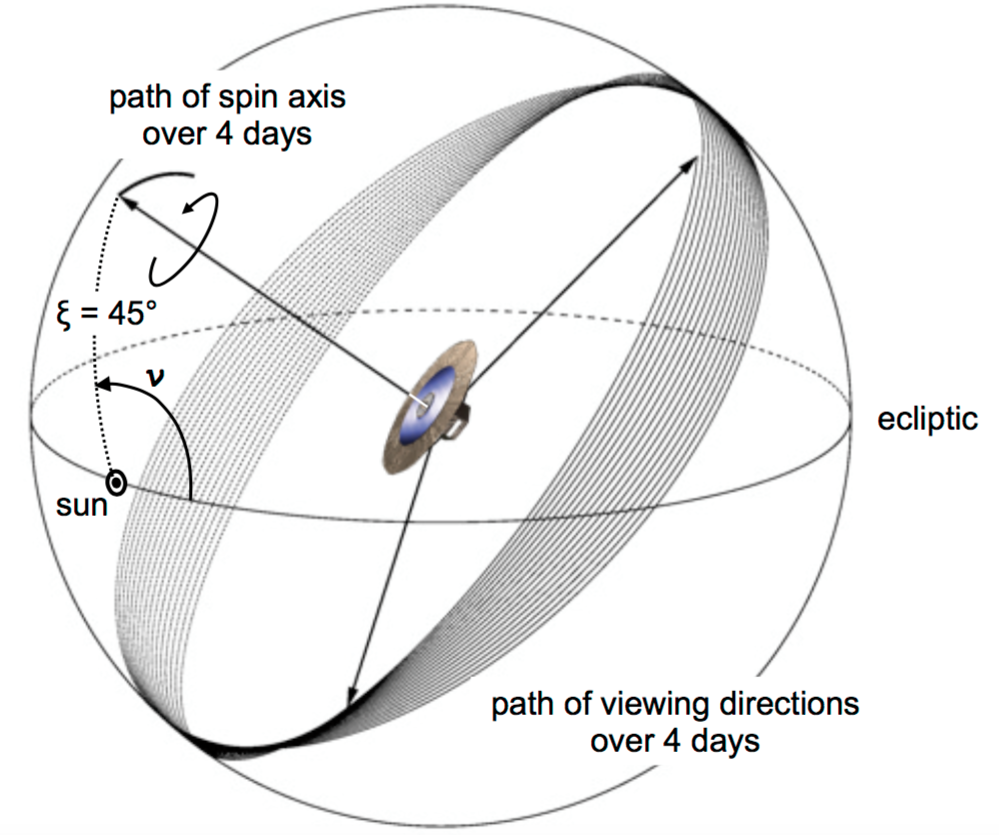# 1.1.4 The scanning law in theory

Author(s): Jos de Bruijne

The scanning law of Gaia determines how the spacecraft scans the sky. It is explained in Gaia Collaboration et al. (2016) and shown schematically in Figure 1.6. The scanning motion consists of three, effectively independent components: a six-hour rotation around the spin axis (at a nominal spin rate of 60 arcsec s${}^{-1}$), a 63-day precession (at a fixed Solar-aspect angle of 45 ${}^{\circ}$) of the spin axis around the Solar direction, and the annual motion of the Earth/L2 with respect to the Sun. This enables full-sky coverage in at least two different scan directions every six months and, on average, 70 focal-plane transits over the nominal, five-year mission.Figure 1.6: Illustration of the scanning law of Gaia, showing the path of the spin axis (z), and the corresponding path of the preceding viewing direction, during four days. For clarity, the path of the following viewing direction is not shown. Figure from Gaia Collaboration et al. (2016).

## Ecliptic-Poles Scanning Law

During the first month of the nominal mission, a special, ecliptic-poles scanning law (EPSL) has been adopted to bootstrap the calibrations needed in the ground-processing software. See Section 1.3.2, Section 4.1, and Gaia Collaboration et al. (2016) for details.

## Nominal Scanning Law

During the remainder of the mission, the nominal scanning law has been used. See Section 1.3.2 and Gaia Collaboration et al. (2016) for details. During the nominal five-year mission, the nominal scanning law results in an average of $\sim$70 usable transits for a given star. It should be noted that, as a result of the scanning law, there is a significant variation of the number of end-of-mission transits as function of position on the sky, in particular as function of ecliptic latitude $\beta$ with regions around $\beta\approx$45 ${}^{\circ}$ being observed more often and regions around the ecliptic poles and plane being observed less frequently. The number 70 assumes that $\sim$20% of the transits collected on board do not produce useful information, for instance as a result of on-board data deletion (Section 1.1.3 and Section 1.3.3). In practice, this is a conservative estimate, in particular for bright stars (Table 1.10).